MORE SITES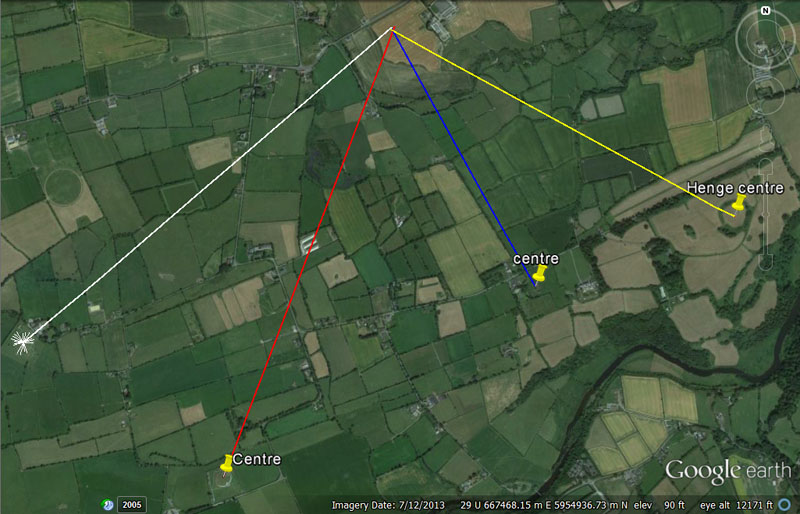Lines running from the 4 centres to the selfsame outer station, form the beginnings of chevrons or lozenge patterns in the landscape, similar to design work that appears on the kerbstones of Newgrange and Knowth chambered mounds, As the assigned, zigzag journeys of individual student groups proceeded from objective to objective, the course might be likened to a chevron pattern across the landscape, as well as, perhaps, an ever expanding lozenge or outwards running spiral that eventually turned and circuited back to the beginning point.

Although much of the kerbstone art is well understood to relate to the movements and phases of the moon, other art might be in allegorical reference to the journeys, out-&-about, of the students studying the navigational arts and using cyclic-astronomy as a tool to locating their list of objectives.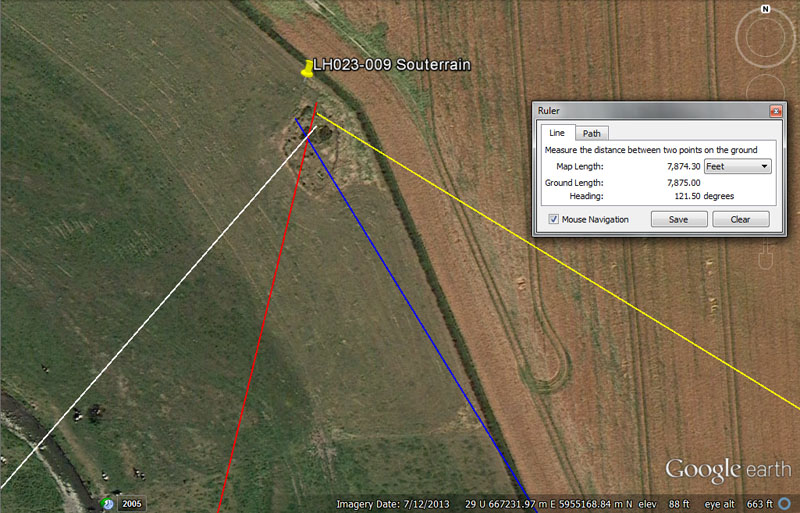Yet more student accommodation was available here at this souterrain complex for the weary orienteers fulfilling their round-&-about navigational assignments.

From Knowth = 8400’ @ 41.66666-degrees.

This distance is, of course, 1.25 ancient Irish miles.

The degree angle is navigational coding and 41.6666-degrees (41 & 2/3rds) is simply 1/3rd of 125-degrees.

From Newgrange = 8910’ @ 14-degrees.

The distance is 1.5 ancient Scottish miles of 5940'.

The degree angle is self-explanatory.

From Dowth Mound = 6250’ @ 148.5-degrees.

The sum of 6.25-miles would be 1/11th of 68.75-miles or 1-degree of arc in the 24750-mile reading of the Earth's circumference.

The angle relates to the 5940' mile of which 1485' would be 1/4th part.

From Dowth Henge = 7875’ @ 121.5-degrees.

This distance is 1.5 miles of 5250'.

The degree angle is navigational also and 1215' would be 1/4th of the 4860' mile, as well as the 1215 value having many applications within lunar cyclic astronomy.

MONKNEWTON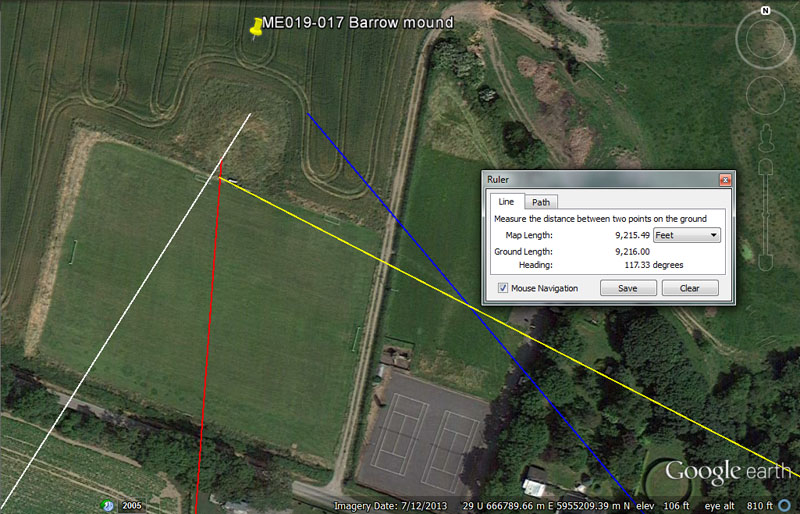Monknewton seems to have been an important centre for transient students, as it offers a number of substantial structures for positional and dimensional analysis and tutorials.

From Knowth = 7680’ @ 32.67-degrees.

The sum of 7680' would be 1/17010st of the 130636800' equatorial circumference. Of course, 1701-days would be 1/4th of the lunar nutation cycle. In the 69.12-mile reading for 1-degree of equatorial arc, the sum of 7.68-miles would be 1/9th part.

The degree angle is navigational also and 3267' would be 1/40000th of the 130680000' equatorial circumference.

From Newgrange = 8800’ @ 4.5-degrees.

The sum of 8800' would be 1 & 2/3rds miles of 5280'.

The degree angle is self explanatory.

From Dowth = 7200’ @ 140-degrees.

This distance is 1/2 a geomancer's mile of 14400'.

The degree angle allows the outrunning vector to skim past the side of Monknewton mound and resolve 35000' from the centre of Dowth onto Rathban Beg Hill, where marker mounds were built.

From Dowth Henge = 9216’ @ 117.3333 @ 118.125-degrees respectively skimming front and rear.

The sum of 921.6-miles would be 1/27th of the 24883.2-mile equatorial circumference.

A degree angle of 117.33333 (117 & 1/3rd) would be 1/45th of the 5280' mile.

The 118.125 vector skims past the rear of the mound and resolves on structures further out. The 118.125 value is lunar coding and 118.125-days would be 4 lunar months or 1/3rd of a lunar year.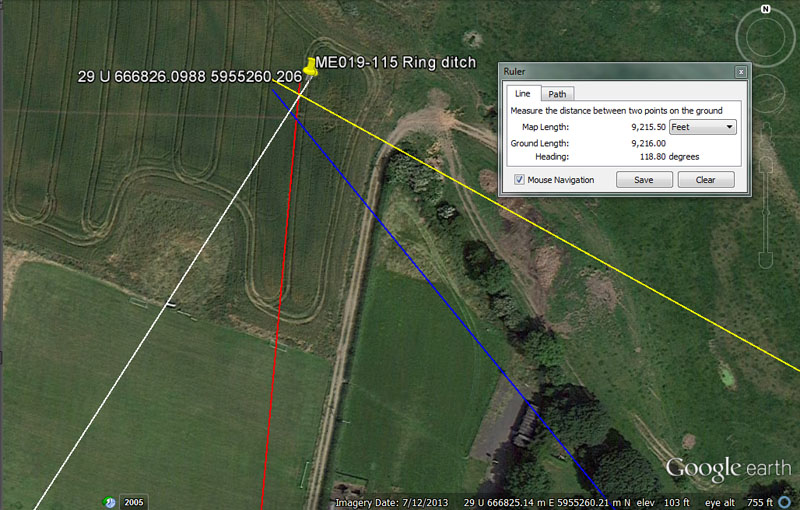The archaeological record places a ring ditch structure at this position.

From Knowth = 7875’ @ 32.67-degrees.

This distance is 1.5-miles of 5250'.

The angle remains the same as the one for the Monknewton mound.

From Newgrange = 9000’ @ 5.25-degrees.

The distance is self explanatory, but if symbolically read as 9072' would be 1.5 minutes of equatorial arc.

The angle is, of course in deference to the 5250' mile.

From Dowth Mound = 7290’ @ 140.8-degrees.

This distance is 1.5 miles of 4860'.

With regards to the degree angle code, the sum of 1408' would be 1/15th of 21120' (4-miles of 5280').

From Dowth Henge = 9187.5’ @ 118.8-degrees.

This distance is 1.75-miles of 5250'.

The degree angle is navigational and 1188' would be 1/5th of 5940' (the ancient Scottish mile).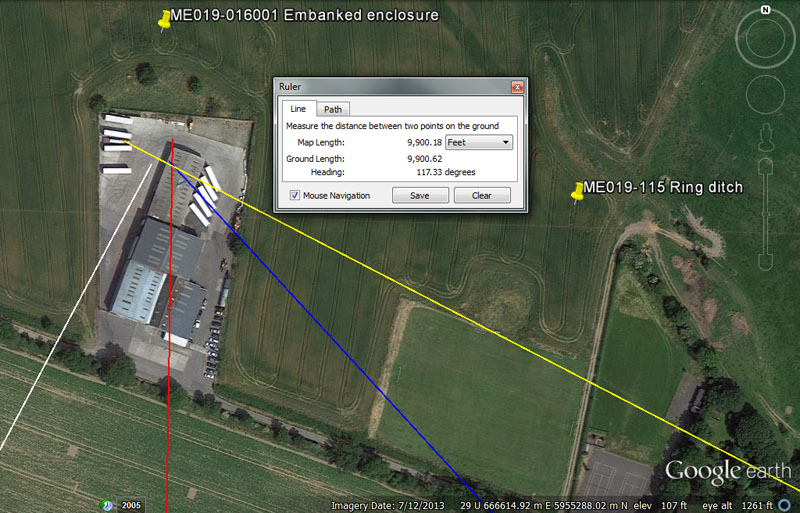A large business and trucking depot has encroached over approximately 2/3rds of this formerly large, embanked enclosure. It was obviously a very important site for the ancient Boyne River Valley open-air-university, as its dynamic codes of position attest.

From Knowth = 7560’ @ 28-degrees.

This distance is 1.25-minutes of equatorial arc.

The degree angle is in homage to the Sabbatical Calendar, where the solar year was allowed to run as 13-months of 28-days each for 7 years before correction.

From Newgrange = 9072’ @ 1-degree.

This distance is 1.5 minutes of equatorial arc.

The 1-degree angle reading is self-explanatory.

From Dowth mound = 7776’ @ 137.5-degrees.

The sum of 777.6-miles would be 1/32nd of the 24883.2-mile equatorial circumference.

The degree angle code is navigational and 13.75-miles would be 1/5th of the 68.75-miles that constituted 1-degree of arc under the 24750-mile reading. Likewise, 1375' = 1/12th of a league of 16500'.

From Dowth Henge = 9900’ @ 118.125 & also 297-degrees (brushing each edge ... as well as the edges of Monknewton Mound) and 117.3333-degrees into the centre section.

This distance is .6 of a league.

Again we have the vectors that brushed Monknewton mound resolving to the centre or brushing each side of this enclosure. An outrunning angle of 297-degrees (half of 594) could also have been accommodated within the confines of this site.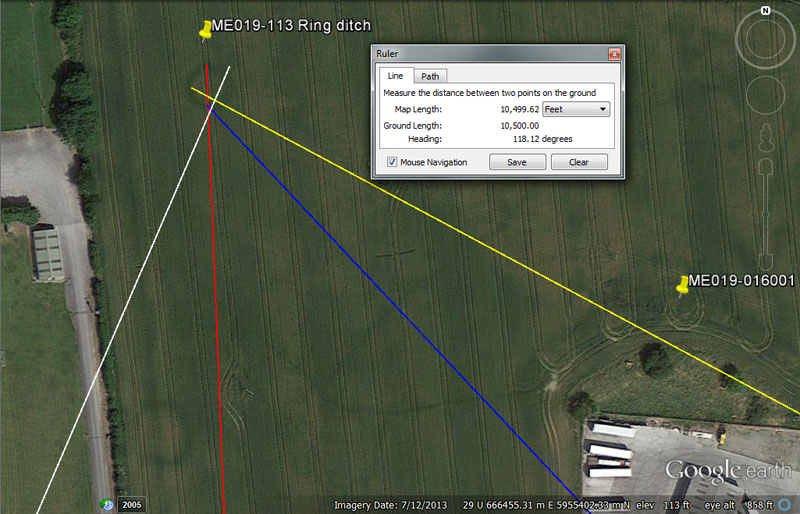Again, there are two ring-ditch crop marks in this field, which seem to have been perfectly circular. They also would have been sized to provide tutorials in converting coded diameters into circumferences divisible by 360-degrees, using special variations on PI.

From Knowth = 7776’ @ 23.328-degrees.

Again, 777.6-miles would be 1/32nd of 24883.2-miles.

The degree angle is in homage to the increment that, over a couple of thousand years later, became the Roman foot (11.664") and 23.328 is twice 11.664. These values were incorporated into ancient volume measures and the Greek Metretes volume for liquids was 2332.8 cubic inches.

From Newgrange = 9504’ @ 177.77777-degrees.

This distance represents a tutorial in converting linear distances into circumferences, using special renditions of PI. Therefore 9504' ÷ 22/7ths = 3024' (half a minute of arc under the 24883.2-miles of 5250' equatorial circumference navigational method). Alternatively, 9504' ÷ 1728/550ths = 3025' (half a minute of arc under the 24750-miles of 5280' equatorial circumference navigational method). This would infer doing legs of travel at sea in either half or full minutes of arc, where the circumference produced was nicely divisible by 360-degrees. Therefore 9504' ÷ 360 = 52.8'. It will be remembered that the Great Pyramid was 3024' in base perimeter value (4 sides of 756'). By symbolically elongating the side length by a mere 3-inches, one circumnavigation became 3025' for half a minute of arc.

The degree angle infers segmenting the 24883.2-mile Earth into 140 divisions, each of 177 & 7/9ths-miles (very slightly rounded). Also, this value X 9 = 1600.

From Knowth = 8437.5’ @ 316.8-degrees.

This distance is lunar coding and 8.4375-days (8 & 7/16ths) would be 1/42nd of a lunar year of 354.375-days. Alternatively, orienteers would locate this site by traveling 8400' (1.25 Irish miles) from Dowth chambered mound.

The degree angle is navigational coding in the same vein as the 9504 value and 9504 ÷ 3 = 3168.

From Dowth Henge = 10500’ @ 118.125-degrees.

This distance is 2-miles of 5250'.

The angle code is lunar and the sum of 118.125-days would equate to 4 lunar months.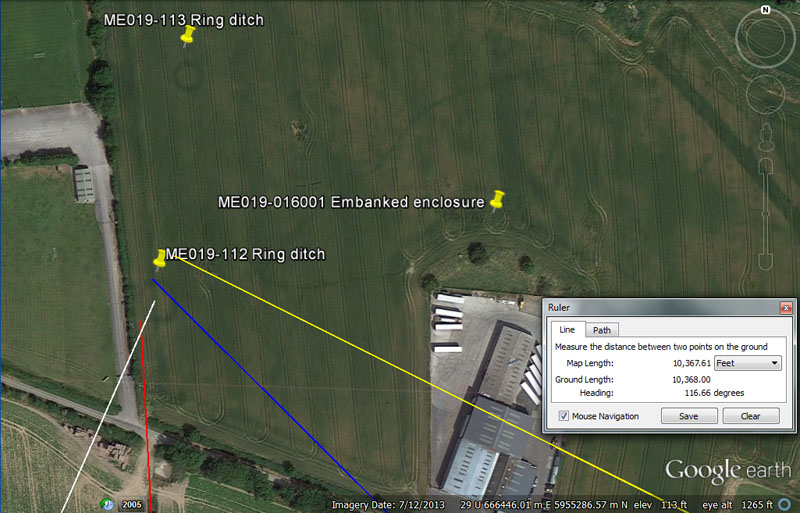Yet another circular ring-ditch which, undoubtedly, offered navigational tutorials in converting linear distances or legs of travel at sea into coded circumferences, fluidly sub-divisible in a 360-degree calculating environment.

From Knowth = 7382.8125’ @ 24-degrees.

This distance is lunar coding and the value 7382.8125 (7382 & 1/16ths) X 4 = 29531.25. The lunar month is 29.53125-days (29 & 17/32nds). The ancient mathematicians obviously memorised values as enlarged, whole numbers before breaking them down into simple or more complex fractions. On the Druidic Calendar of Coligny brass plaque both lunar and solar periods are displayed over a 1890-day* calculation period. If one uses the in-built facility to divide the 1890-day period by 63, then that equates to 30-days for the solar month count. If one divides the 1890-day period by a secondary in-built facility of 64, then that equates to 29.53125-days, the duration of a lunar month to a tolerance of 1-minute in time.
*Note: the 756' length of the Great Pyramid is 189' X 4.

The 24-degree angle code is pretty much self-explanatory and 24-degrees would be 1/15th of a 360-degree circle or there are 24-hours in a day, etc.

From Newgrange = 9072’ @ 177.1875-degrees.

This distance is 1.5-minutes of arc.

The degree angle is lunar coding and the sum of 177.1875-days (177 & 3/16ths) is half a lunar year of 354.375-days.

From Dowth Mound = 8250’ @ 135-degrees.

The distance of 8250' is half a league of 16500'.

The 135-degrees angle is both lunar and navigational coding simultaneously and the value 135, in a mathematical progression, produces a string of numbers applicable to both. Twice 135-degrees is 270-degrees and the sum of 135-degrees would be 12/32nds (3/8ths) of 360-degrees.

From Dowth Henge = 10368 @ 116.64-degrees. The degree angle sweeps a bit high, but was still sufficient for the orienteers to arrive at and identify the site on that vector.

This value is for mnemonic recall of how fast the Earth spins in 24-hours. Therefore, 24883.2-miles ÷ 24-hours = 1036.8 MPH.

The angle (which can also be rendered more simply as 116 & 2/3rds) is in homage to the so-called Roman foot of 11.664".

Continue